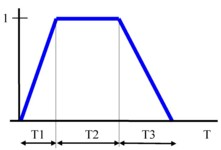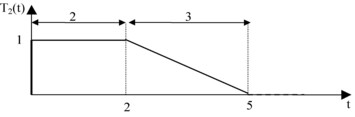# Trapez function

## Interest

The Trapez function allows creating signals of a trapezoidal shape.

## Definition

The Trapez(X,X1,X2,X3) function returns:

• 1, if X is in [X1,X1+X2]
• 0, if X is in ]-∞,0] or [X1+X2+X3, +∞]
• an interpolated value between 0 and 1

if X is in [0, X1] or [X1+X2, X1+X2+X3].

Note: The arguments X, X1 , X2 et X3 can be formulas.

## Operation mode

In case of transient application it is possible to define the trapezoidal pulse function shown in the figure below.

Create an I/O parameter: PE = Trapez(TIME,T1,T2,T3)

where:

• TIME is the time parameter
• T1 is the time interval of linear increase of the function
• T2 is the time interval of constant value of the function
• T3 is the time interval of linear decrease of the function## Example

An example of the Trapez function is presented below.

Trapeze function: T2(t) = Trapez(t,0,2,3)